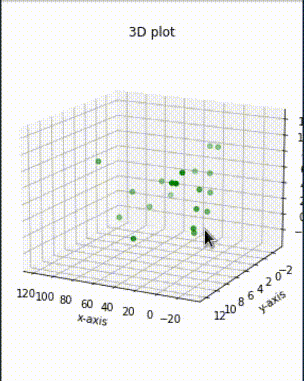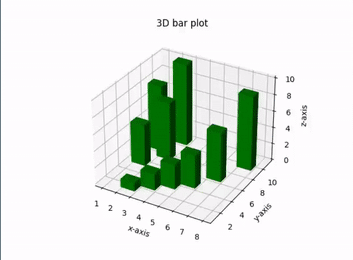# Make 3D interactive Matplotlib plot in Jupyter Notebook

Matplotlib is a data visualization library. It is difficult to analyze/get an insight into the data without visualizing it. And matplotlib is a great library for doing the visual analysis of data in python. Using matplotlib we can plot 1-D, 2-D and even 3-D  data. In this article, we are going to learn how we can plot various 3-D plots using the matplotlib. To plot 3-D plots in python, we need to import the mplot3d library from the standard installation of matplotlib library from python.

As matplotlib is a third-party library, it doesn’t come with a standard installation of python, so you need to install matplotlib before following this article. Below is the command using which you can install the matplotlib library.

Syntax:

pip3 install matplotlib

To make the plots interactive all you need to do is install another library called ipympl i.e. interactive python matplotlib.

Syntax:

pip3 install ipympl

For creating 3d figure Axes3D.plot() function is used.

Syntax:

Axes3D.plot(xs, ys, zs,*args, **kwargs)

Parameter:

• xs: the x coordinate value of the vertices.
• ys: the y coordinate values of the vertices.
• zs: The z coordinate value(s), either one for all points or one for each point.

To generate an interactive 3D plot first import the necessary packages and create a random dataset. Now using Axes3D(figure)  function from the mplot3d library we can generate a required plot directly. Pass the data to the 3D plot and configure the title and labels.

Note: We must needed to add ” %matplotlib widget  “, it is a Jupyter magic widget and used to tell jupyter to use interactive backend for plot. We have to add it to the top of the script to create an interactive plot in the ipython notebook (i.e. Jupyter notebook, Google Colab, Kaggle Kernel, etc.) to renders the figure as an interactive figure.

Given below are some implementations to do the same.

Example: Interactive scatter plot

## Python3

 `# creating 3d plot using matplotlib ` `# in python`   `# for creating a responsive plot` `%``matplotlib widget`   `# importing required libraries` `from` `mpl_toolkits.mplot3d ``import` `Axes3D` `import` `matplotlib.pyplot as plt`   `# creating random dataset` `xs ``=` `[``14``, ``24``, ``43``, ``47``, ``54``, ``66``, ``74``, ``89``, ``12``,` `      ``44``, ``1``, ``2``, ``3``, ``4``, ``5``, ``9``, ``8``, ``7``, ``6``, ``5``]`   `ys ``=` `[``0``, ``1``, ``2``, ``3``, ``4``, ``5``, ``6``, ``7``, ``8``, ``9``, ``6``, ``3``,` `      ``5``, ``2``, ``4``, ``1``, ``8``, ``7``, ``0``, ``5``]`   `zs ``=` `[``9``, ``6``, ``3``, ``5``, ``2``, ``4``, ``1``, ``8``, ``7``, ``0``, ``1``, ``2``, ` `      ``3``, ``4``, ``5``, ``6``, ``7``, ``8``, ``9``, ``0``]`   `# creating figure` `fig ``=` `plt.figure()` `ax ``=` `Axes3D(fig)`   `# creating the plot` `plot_geeks ``=` `ax.scatter(xs, ys, zs, color``=``'green'``)`   `# setting title and labels` `ax.set_title(``"3D plot"``)` `ax.set_xlabel(``'x-axis'``)` `ax.set_ylabel(``'y-axis'``)` `ax.set_zlabel(``'z-axis'``)`   `# displaying the plot` `plt.show()`

Output:Interactive 3D plot in Jupyter notebook

Example: Interactive bar plot

## Python

 `# creating 3d bar plot using matplotlib ` `# in python`   `# to interact  with plot` `%``matplotlib widget`   `# importing required libraries` `from` `mpl_toolkits.mplot3d ``import` `Axes3D` `import` `matplotlib.pyplot as plt` `import` `numpy as np`   `# creating random dataset` `xs ``=` `[``2``, ``3``, ``4``, ``5``, ``1``, ``6``, ``2``, ``1``, ``7``, ``2``]` `ys ``=` `[``1``, ``2``, ``3``, ``4``, ``5``, ``6``, ``7``, ``8``, ``9``, ``10``]` `zs ``=` `np.zeros(``10``)` `dx ``=` `np.ones(``10``)` `dy ``=` `np.ones(``10``)` `dz ``=` `[``1``, ``2``, ``3``, ``4``, ``5``, ``6``, ``7``, ``8``, ``9``, ``10``]`   `# creating figure` `figg ``=` `plt.figure()` `ax ``=` `figg.add_subplot(``111``, projection``=``'3d'``)`   `# creating the plot` `plot_geeks ``=` `ax.bar3d(xs, ys, zs, dx, ` `                      ``dy, dz, color``=``'green'``)`   `# setting title and labels` `ax.set_title(``"3D bar plot"``)` `ax.set_xlabel(``'x-axis'``)` `ax.set_ylabel(``'y-axis'``)` `ax.set_zlabel(``'z-axis'``)`   `# displaying the plot` `plt.show()`

Output:Interactive 3D plot

Whether you're preparing for your first job interview or aiming to upskill in this ever-evolving tech landscape, GeeksforGeeks Courses are your key to success. We provide top-quality content at affordable prices, all geared towards accelerating your growth in a time-bound manner. Join the millions we've already empowered, and we're here to do the same for you. Don't miss out - check it out now!